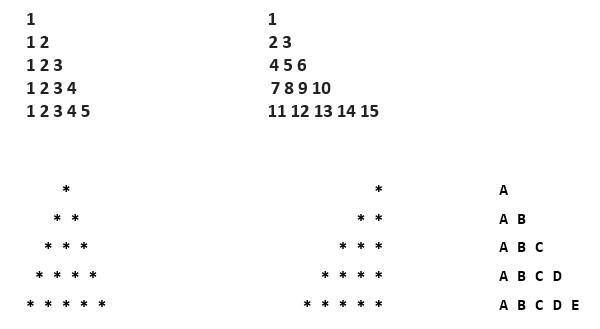# How to print pattern in Python?

PythonServer Side ProgrammingProgramming

Patterns in Python can be printed using nested for loops. The outer loop is used to iterate through the number of rows whereas the inner loop is used to handle the number of columns. The print statement is modified to form various patterns according to the requirement.

The patterns can be star patterns, number patterns, alphabet patterns. The patterns can be of different shapes, triangle, pyramids, etc.

### ExampleAll these patterns can be printed with the help of for loops with modified print statements which forms these different patterns.

The basic idea between the printing of these patterns is the same with slight differences.

We will implement the code for some of these patterns.

## Printing Triangle

### Example

def triangle(n):
k=n-1
for i in range(1,n+1):
for j in range(k):
print(" ",end="")
k=k-1
for p in range(i):
print("*",end=" ")
print()
print("Enter number of rows")
r=int(input())
triangle(r)

### Output

Enter number of rows
5
*
* *
* * *
* * * *
* * * * *

Let us try running the above code with different number of rows −

## Number pattern

### Example

def num_pattern(n):
num=1
for i in range(1,n+1):
for j in range(i):
print(num,end=" ")
num+=1
print()
print("Enter number of rows")
r=int(input())
num_pattern(r)

### Output

Enter number of rows
5
1
2 3
4 5 6
7 8 9 10
11 12 13 14 15

## Alphabet pattern

### Example

def alpha_pattern(n):
st="ABCDEFGHIJKLMNOPQRSTUVWXYZ"
for i in range(1,n+1):
for j in range(i):
print(st[j],end=" ")
print()
print("Enter number of rows")
r=int(input())
alpha_pattern(r)

### Output

Enter number of rows
5
A
A B
A B C
A B C D
A B C D E

## Pyramid (rotated 180 degree)

### Example

def pyramid(n):
k=n-1
for i in range(1,n+1):
for j in range(k):
print(" ",end="")
for p in range(i):
print("*",end=" ")
k=k-1
print()
print("Enter number of rows")
r=int(input())
pyramid(r)

### Output

Enter number of rows
5
*
* *
* * *
* * * *
* * * * *

Run the above code on IDE to get an accurate view of the pattern.